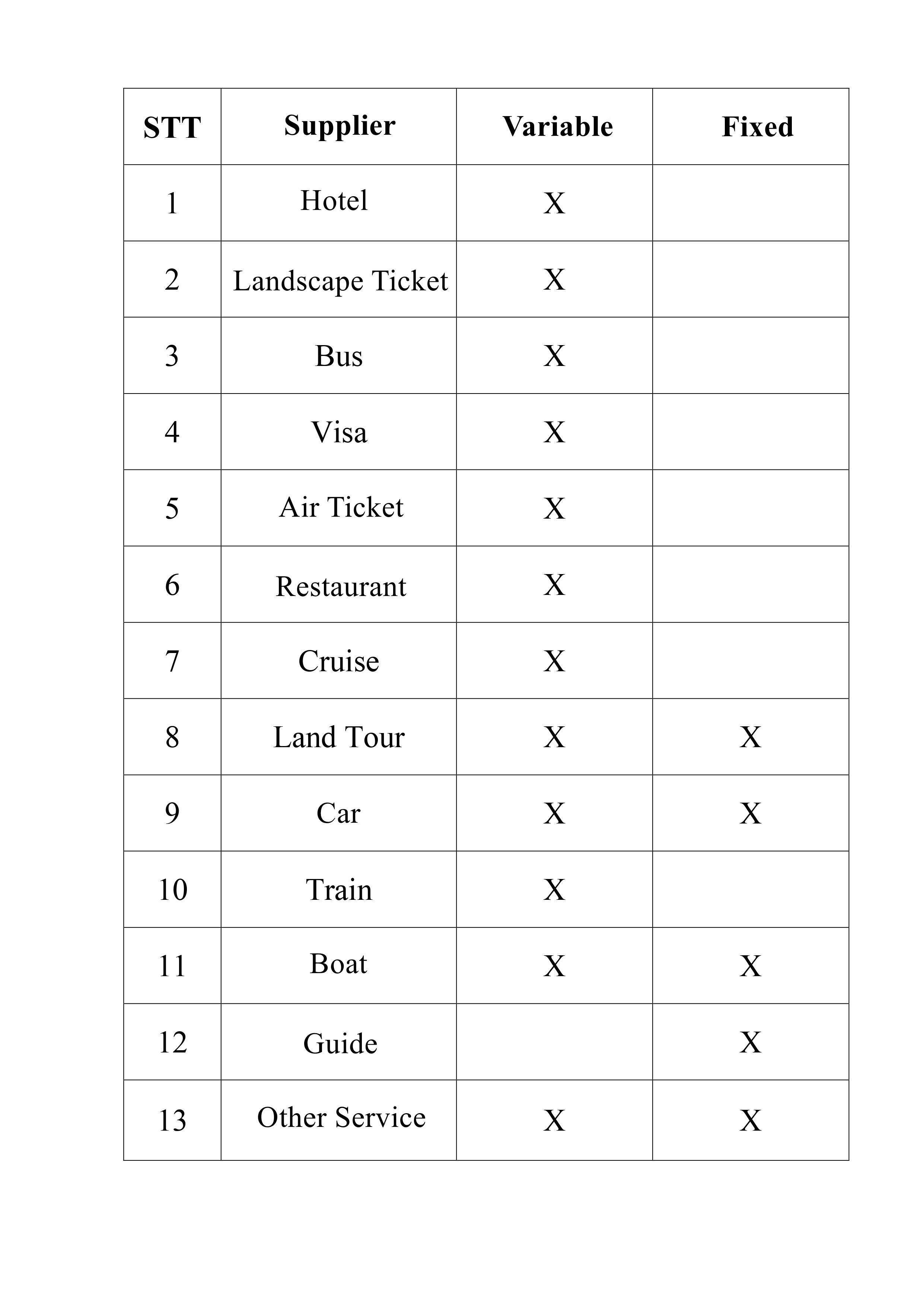# Variable Price/ Fixed Price

What is variable price? Is a term often used in the calculation of tour groups with a service that is proportional to the number of tourists using that service.What is variable price? Is a term often used in the calculation of tour groups with a service that is proportional to the number of tourists using that service.

With each service has its own characteristics, it can be applied to variable or fixed price depending on the operator's calculation, but when applying TravelMaster software, the principle for Fixed Price - Variable is defined as:In order to be defined in software Travel Master, the system has integrated double operations to pre-calculate to help the operator to avoid price deviation when applied in the system.

1. Hotel: With the calculation of 2 pax/Room in the product tour, the 3rd guest is counted = 1/2 of the first Room, the 4th guest is counted = 2 Room

2. Landscape Ticket: The number of guests is proportional to the number of tickets

3. Bus: The number of guests is proportional to the number of tickets

4. Visa: The number of guests is proportional to the number of tickets

5. Air Ticket: The number of guests is proportional to the number of tickets

6. Restaurant: Calculated according to the set menu, so the number of guests will be proportional to the number of sets to be ordered at the restaurant/per meal

7. Cruise: The number of guests is proportional to the number of seat in cabin

8. Land tour: The number of guests is proportional to the number of tickets

9. Car: The number of cars booked can be directly proportional (variable), inversely proportional (fixed) to the number of passengers

10. Train: The number of guests is proportional to the number of tickets

11. Boat: The number of boats can be proportional (variable), inversely proportional (fixed) to the number of passengers (note: Boat is currently defined as a vessel traveling on a river, for example: Saigon River, Nho Que river)

12. Guide: The number of guides can be proportional (variable), inversely proportional (fixed) to the number of guests